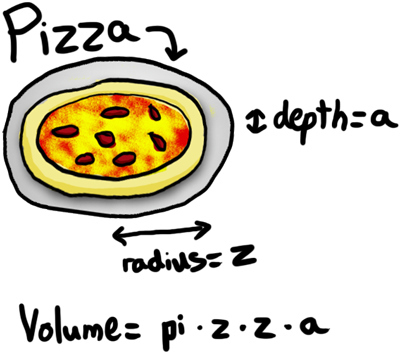# Courses

### Courses Taught

Honors Calculus 1
Calculus 2
Calculus 3
Differential Equations
Complex Variable
Euclidean Geometry
Geometry
Topology
Linear Algebra
Computational Linear Algebra
Numerical Linear Algebra
Abstract Algebra
Fractal Geometry
Tilings and Fractals
Discrete Mathematics
Graph Theory
Combinatorics 1
Combinatorics 2
Enumeration and Graphs
Codes and Designs
Combinatorial Geometry
Computational Geometry
Probability
Stochastic Processes